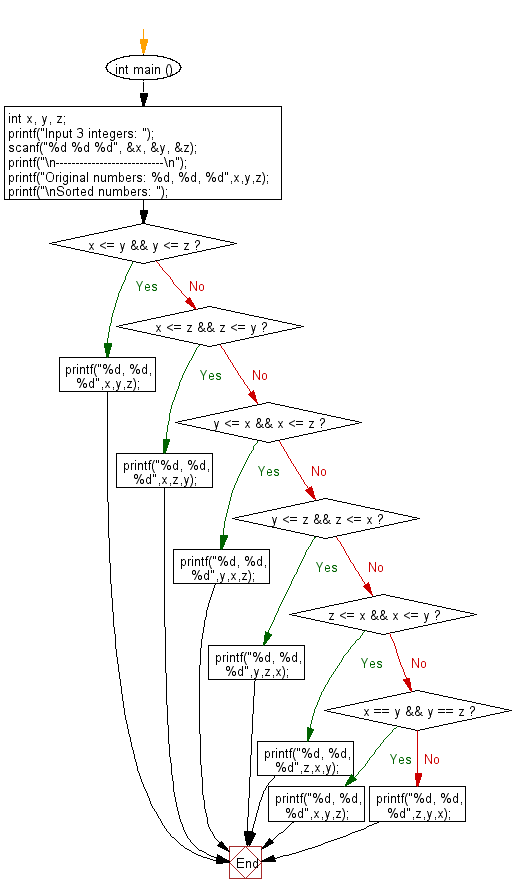﻿ C : Read 3 integers and sort the numbers in ascending order

# C Exercises: Read three integers and sort the numbers in ascending order

## C Basic Declarations and Expressions: Exercise-102 with Solution

Write a C program that reads three integers and sorts the numbers in ascending order. Print the original numbers and the sorted numbers.

Sample Solution:

C Code:

``````#include <stdio.h>
#include <math.h>

int main (){
// Declare three integer variables
int x, y, z;

// Prompt the user to input three integers
printf("Input 3 integers: ");

// Read the input values for x, y, and z
scanf("%d %d %d", &x, &y, &z);

// Print a separator line for clarity
printf("\n---------------------------\n");

// Display the original input numbers
printf("Original numbers: %d, %d, %d", x, y, z);

// Display the sorted numbers
printf("\nSorted numbers: ");

// Nested if-else statements to sort and print the numbers
if (x <= y && y <= z){
printf("%d, %d, %d", x, y, z);
}
else{
if (x <= z && z <= y){
printf("%d, %d, %d", x, z, y);
}
else{
if (y <= x && x <= z){
printf("%d, %d, %d", y, x, z);
}
else{
if (y <= z && z <= x){
printf("%d, %d, %d", y, z, x);
}
else{
if (z <= x && x <= y){
printf("%d, %d, %d", z, x, y);
}
else{
if (x == y && y == z){
printf("%d, %d, %d", x, y, z);
}
else{
printf("%d, %d, %d", z, y, x);
}
}
}
}
}
}
}
``````

Sample Output:

```Input 3 integers: 17
-5
25

---------------------------
Original numbers: 17, -5, 25
Sorted numbers: -5, 17, 25
```

Flowchart:C programming Code Editor:

What is the difficulty level of this exercise?

Test your Programming skills with w3resource's quiz.

﻿